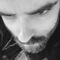# Variable multiplier inputs based on another selectRobin Martinez
Member | 81
+
0
-

Hello, I am using contributte/forms-multiplier and have following question:

Let's say I have a form select. When I select item A, I'd like multiplier to have single input, let's say:

``````		\$multiplier = \$c->addMultiplier('values', function (\Nette\Forms\Container \$container, \Nette\Forms\Form \$form) {
}, 0, 20);``````

but when I select item B in form select, I'd like multiplier to show me two inputs, let's say:

``````		\$multiplier = \$c->addMultiplier('values', function (\Nette\Forms\Container \$container, \Nette\Forms\Form \$form) {
}, 0, 20);``````

Is this somehow possible? Or is there another (better) solution to achieve something simiar?

Thank you!Martk
Member | 648
+
0
-

https://github.com/…doc/index.md

But I don't know if it works with multiplierRobin Martinez
Member | 81
+
0
-

Yeah, I actually use that component as well, but as you mentioned, I also don't think it will work… :/Robin Martinez
Member | 81
+
0
-

Okay, the solution for me is to show both fields and make the second ‘requireable’ as necessary via addRule()